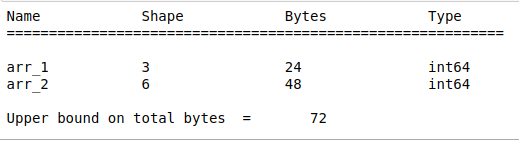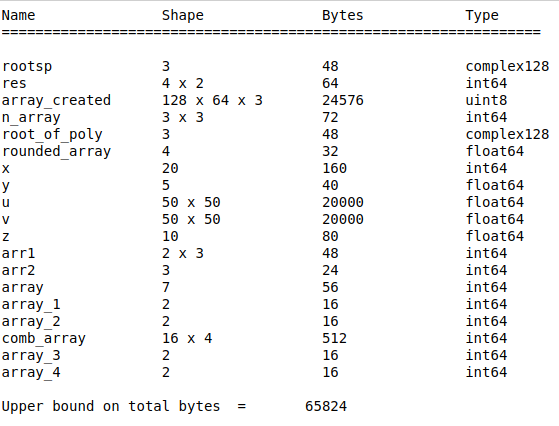Open in App
Not now

# numpy.who() function in Python

• Last Updated : 05 Sep, 2020

numpy.who(vardict=None) function prints the Numpy ndarrays in the given dictionary. If there is no dictionary passed in or vardict is None then prints NumPy arrays in the globals() dictionary.

Parameters:

vardict: A dictionary possibly containing ndarrays. Default is globals().

Returns:

out: None

Note: It prints out the name, shape, bytes and type of all of the ndarrays present in vardict but returns none.

Example #1: In this example a dictionary is passed as argument to the numpy.who() function.

## Python3

 `# import the numpy module as np``import` `numpy as np`` ` `# dictionary containing numpy ndarrays``gfg ``=` `{``'arr_1'``: np.arange(``3``), ``'arr_2'``: np.arange(``6``),``       ``'name'``: ``'some text'``, ``'number'``: ``34523``}`` ` `# passing the dict as argument``np.who(gfg)`

Output:Example #2: In this example no argument is passed to the numpy.who() function so it prints ndarray in globals() dictionary.

## Python3

 `# import the numpy module as np``import` `numpy as np`` ` `# creating numpy ndarrays``x ``=` `np.arange(``20``)``y ``=` `np.ones(``5``)``z ``=` `np.zeros(``10``)`` ` `# function called without passing any argument``np.who()`

Output:My Personal Notes arrow_drop_up